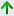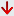# Pandas Exercises, Practice, Solution

## Pandas

pandas is a Python package providing fast, flexible, and expressive data structures designed to make working with 'relationa' or 'labeled' data both easy and intuitive. It aims to be the fundamental high-level building block for doing practical, real world data analysis in Python.pandas is well suited for many different kinds of data:

• Tabular data with heterogeneously-typed columns, as in an SQL table or Excel spreadsheet
• Ordered and unordered (not necessarily fixed-frequency) time series data.
• Arbitrary matrix data with row and column labels
• Any other form of observational / statistical data sets.

Binary Installers: https://pypi.org/project/pandas

Source Repository: http://github.com/pandas-dev/pandas

Issues & Ideas: https://github.com/pandas-dev/pandas/issues

Pandas Basic commands:

Imports the following commands to start:

```import pandas as pd
import numpy as np```

Pandas version:

```import pandas as pd
print(pd.__version__)
```
 Key and Imports df pandas DataFrame object s pandas Series object

Create Dataframe:

``````import pandas as pd
df = pd.DataFrame({'X':[78,85,96,80,86], 'Y':[84,94,89,83,86],'Z':[86,97,96,72,83]});
print(df)
``````

Sample Output:

```    X   Y   Z
0  78  84  86
1  85  94  97
2  96  89  96
3  80  83  72
4  86  86  83
```

Create DataSeries:

``````import pandas as pd
s = pd.Series([2, 4, 6, 8, 10])
print(s)
``````

Sample Output:

```0     2
1     4
2     6
3     8
4    10
dtype: int64
```

Create Test Objects

 pd.DataFrame(np.random.rand(20,5)) 5 columns and 20 rows of random floats pd.Series(my_list) Create a series from an iterable my_list df.index = pd.date_range('1900/1/30', periods=df.shape) Add a date index

Viewing/Inspecting Data

 df.head(n) First n rows of the DataFrame df.tail(n) Last n rows of the DataFrame df.shape Number of rows and columns df.info() Index, Datatype and Memory information df.describe() Summary statistics for numerical columns s.value_counts(dropna=False) View unique values and counts df.apply(pd.Series.value_counts) Unique values and counts for all columns

Selection

 df[col] Returns column with label col as Series df[[col1, col2]] Returns columns as a new DataFrame s.iloc Selection by position s.loc['index_one'] Selection by index df.iloc[0,:] First row df.iloc[0,0] First element of first column

Data Cleaning

 df.columns = ['a','b','c'] Rename columns pd.isnull() Checks for null Values, Returns Boolean Arrray pd.notnull() Opposite of pd.isnull() df.dropna() Drop all rows that contain null values df.dropna(axis=1) Drop all columns that contain null values df.dropna(axis=1,thresh=n) Drop all rows have have less than n non null values df.fillna(x) Replace all null values with x s.fillna(s.mean()) Replace all null values with the mean s.astype(float) Convert the datatype of the series to float s.replace(1,'one') Replace all values equal to 1 with 'one' s.replace([2,3],['two', 'three']) Replace all 2 with 'two' and 3 with 'three' df.rename(columns=lambda x: x + 1) Mass renaming of columns df.rename(columns={'old_name': 'new_ name'}) Selective renaming df.set_index('column_one') Change the index df.rename(index=lambda x: x + 1) Mass renaming of index

Filter, Sort, and Groupby

 df[df[col] > 0.6] Rows where the column col is greater than 0.6 df[(df[col] > 0.6) & (df[col] < 0.8)] Rows where 0.8 > col > 0.6 df.sort_values(col1) Sort values by col1 in ascending order df.sort_values(col2,ascending=False) Sort values by col2 in descending order.5 df.sort_values([col1,col2],ascending=[True,False]) Sort values by col1 in ascending order then col2 in descending order df.groupby(col) Returns a groupby object for values from one column df.groupby([col1,col2]) Returns groupby object for values from multiple columns df.groupby(col1)[col2] Returns the mean of the values in col2, grouped by the values in col1 df.pivot_table(index=col1,values=[col2,col3],aggfunc=mean) Create a pivot table that groups by col1 and calculates the mean of col2 and col3 df.groupby(col1).agg(np.mean) Find the average across all columns for every unique col1 group df.apply(np.mean) Apply the function np.mean() across each column nf.apply(np.max,axis=1) Apply the function np.max() across each row

Join/Combine

 df1.append(df2) Add the rows in df1 to the end of df2 (columns should be identical) pd.concat([df1, df2],axis=1) Add the columns in df1 to the end of df2 (rows should be identical) df1.join(df2,on=col1, how='inner') SQL-style join the columns in df1 with the columns on df2 where the rows for col have identical values. The 'how' can be 'left', 'right', 'outer' or 'inner'

Statistics

 df.describe() Summary statistics for numerical columns df.mean() Returns the mean of all columns df.corr() Returns the correlation between columns in a DataFrame df.count() Returns the number of non-null values in each DataFrame column df.max() Returns the highest value in each column df.min() Returns the lowest value in each column df.median() Returns the median of each column df.std() Returns the standard deviation of each column

Importing Data

Exporting Data

 df.to_csv(filename) Write to a CSV file df.to_excel(filename) Write to an Excel file df.to_sql(table_name, connection_object) Write to a SQL table df.to_json(filename) Write to a file in JSON format

List of Exercises with Solutions :

## Popularity of Programming Language Worldwide, Aug 2020 compared to a year ago:

Rank Change Language Share Trend
1 Python 31.59 % +3.3 %
2 Java 16.9 % -2.7 %
3 Javascript 8.17 % +0.0 %
4 C# 6.54 % -0.7%
5C/C++ 5.88 % +0.1 %
6PHP 5.78 % -0.7 %
7 R 4.18 % +0.3 %
8 Objective-C 2.6 % -0.0 %
9 Swift 2.35 % -0.0 %
10TypeScript 1.94% +0.2 %
11Matlab 1.63 % -0.2 %
12   Kotlin 1.57 % +0.1 %
13Go 1.39 % +0.2 %
14Ruby 1.22 % -0.2 %
15VBA 1.19 % -0.1 %
16 Scala 0.97 % -0.1 %
17Rust 0.91 % +0.3 %
18Visual Basic 0.82 % -0.2 %
19Dart 0.57 % +0.2 %
20Ada 0.54 % +0.2 %
21Lua 0.52 % +0.1 %
22Perl 0.45 % -0.1 %
23Abap 0.44 % -0.1 %
24Julia 0.43 % +0.2 %
25   Cobol 0.42 % +0.1 %
26Groovy 0.41 % -0.1 %
27Haskell 0.32 % +0.0 %
28 Delphi 0.28 % +0.0 %

Source : http://pypl.github.io/PYPL.html

TIOBE Index for August 2020

Aug 2020 Aug 2019 Change Programming Language Ratings Change
1 2C 16.98% +1.83%
2 1Java 14.43% -1.60%
3 3 Python 9.69% -0.33%
4 4 C++ 6.84% +0.78%
5 5 C# 4.68% +0.83%
6 6 Visual Basic 4.66% +0.97%
7 7 JavaScript 2.87% +0.62%
8 20R 2.79% +1.97%
9 8PHP 2.24% +0.17%
10 10 SQL 1.46% -0.17%
11 17Go 1.43% +0.45%
12 18Swift 1.42% +0.53%
13 19Perl 1.11% +0.25%
14 15Assembly language 1.04% -0.07%
15 11Ruby 1.03% -0.28%
16 12MATLAB 0.86% -0.41%
17 16Classic Visual Basic 0.82% -0.20%
18 13Groovy 0.77% -0.46%
19 9Objective-C 0.76% -0.93%
20 28Rust 0.74% +0.29%

Source : https://www.tiobe.com/tiobe-index/

[ Want to contribute to Python Pandas exercises? Send your code (attached with a .zip file) to us at w3resource[at]yahoo[dot]com. Please avoid copyrighted materials.]

Test your Python skills with w3resource's quiz

﻿

## Python: Tips of the Day

Python: Use Enumerate() In for Loops

```>>> students = ('John', 'Mary',  'Mike')
>>> for i, student in enumerate(students):
...     print(f'Iteration:  {i}, Student: {student}')
...
Iteration: 0, Student: John
Iteration: 1, Student: Mary
Iteration: 2, Student: Mike
>>> for i, student in enumerate(students,  35001):
...      print(f'Student Name: {student}, Student ID #: {i}')
...
Student Name: John, Student ID #: 35001
Student Name: Mary, Student ID #: 35002
Student Name: Mike, Student ID #: 35003
```